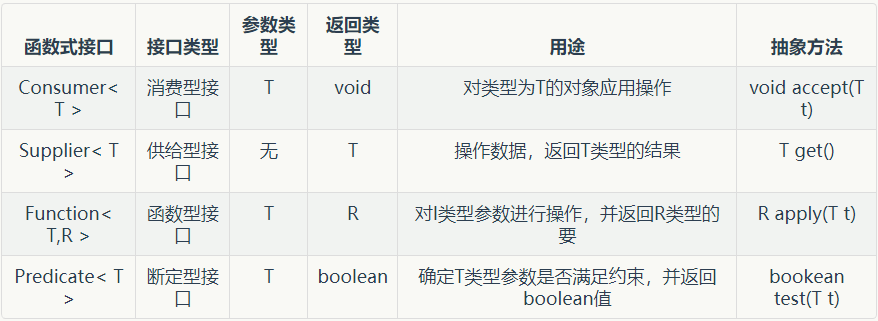# 什么是函数式接口

函数式接口是Java8引用的一个新特性，是一种特殊的接口:SAM类型的接口（Single Abstract Method）。但是它还是一个接口，只是有些特殊罢了。  函数式接口的出现主要是为了配合Java8的另一个新特性Lamdba表达式来使用。

• 接口中只有一个抽象方法
• 接口中面可以加一个注解@FunctionalInterface来检查接口中的方法是不是只有一个抽象方法
• 在接口里面可以加入 默认方法静态方法
• 函数式接口也可以继承，但是继承的时候，抽象方法必须一样
• 函数式接口重写父类的的方法，并不会计入到自己的抽象方法中

# 自定义函数式接口

``````//加入这个注解是为了检测接口中是否符合函数式接口的要求
@FunctionalInterface
public interface MyFunctionInterction {
//唯一的抽象方法
void absoluMethod();

//重写Object的方法
@Override
String toString();

//默认方法
default void defaultMethod() {
System.out.println("默认方法");
}

//静态方法
static void stativMethod() {
System.out.println("静态方法");
}
}``````

# 函数式接口的简单使用

``里面的默认方法可以直接使用``
``````public class TestFunctionIntection {
public static void main(String[] args) {
TestFunctionIntection testFunctionIntection =
new TestFunctionIntection();
testFunctionIntection.test(
//Lamdba表达式的简单使用
() -> System.out.println("函数式接口里面的抽象方法"));
}

/**
* 自己定义的一个方法，并使用自定义的一个消费类型的函数式接口
* @param myFunctionInterction
*/
public void test(MyFunctionInterction
myFunctionInterction) {

//函数式接口里面的抽象方法
myFunctionInterction.absoluMethod();

//默认方法
myFunctionInterction.defaultMethod();

//静态方法
MyFunctionInterction.stativMethod();
}
}``````

# java8里面自定义的四个核心的函数式接口# 下面是这四个核心接口的简单使用

``````public class FunctionTest {
//Consumer<T> 消费型接口
@Test
public void test1() {
Consumer<String> consumer = (x) -> System.out.println(x);
consumer.accept("消费型接口，没有返回值！");
}
//输出：消费型接口，没有返回值！

//供给型接口
@Test
public void test2() {
Supplier<String> supplier = () -> "主要的作用就是创建对象！";
String s = supplier.get();
System.out.println(s);
}
//输出：主要的作用就是创建对象！

//函数型接口
//Function<T,R> T 接收的参数，R 返回值类型
@Test
public void test3() {
Function<Integer, String> function = (x) -> x + "：为String类型";
String apply = function.apply(7);
System.out.println(apply);
}
//输出：7:为String类型

//断言型接口
@Test
public void test4() {
Predicate<Integer> predicate = (x) -> x > 10;
boolean test = predicate.test(11);
System.out.println(test);
}
//输出：true
}``````

## Consumer 的应用

``````//Consumer<T> 消费型接口
@Test
public void test1() {
//定义一个消费型接口，只输出输入的内容
Consumer<String> consumer = (x) -> System.out.println(x);
//在输入的内容后面加上·－－加上了默认方法·
Consumer<String> consumer2 = (x) -> System.out.println(x + "－－加上了默认方法");
consumer.andThen(consumer2).accept("消费型接口，没有返回值！");
}
//输出：消费型接口，没有返回值                     (accept输出的值）

Consumer 的默认方法的源码：

``````default Consumer<T> andThen(Consumer<? super T> after) {
Objects.requireNonNull(after);
return (T t) -> { accept(t); after.accept(t); };
}``````

其返回值这一句是重点，先是传入一个Consumer接口，然后返回一个Consumer接口，说明可以用表达式链，然后用这个特性可以把数据进一次进行加工。
(T t) -> { accept(t); after.accept(t); };这一句，返回的顺序首先是调用抽象方法，然后再调用默认方法，说明这个默认方法只可以对数据进行再加工，不能再抽象方法前面。

## Supplier 的应用

``````    //供给型接口，这个方法若以用在工厂方法中
@Test
public void test2() {
//跟据一个字符串创建对象
Supplier<String> supplier = () -> "主要的作用就是创建对象！";
//获取一个对象
String s = supplier.get();
//获取两个以象
String s1 = supplier.get();
//两个对象内容一样
System.out.println(s.equals(s1));
System.out.println(s);
//用方法引用的方式创建一个对象
Supplier<SupplierTest> testSupplier = SupplierTest::new;
//用new的方式创建一个对象
Supplier<SupplierTest> supplierTestSupplier = () -> new SupplierTest("张三");
//可以通过supplierTestSupplier 来获取一个对象，并且可以调用里面的方法
String name = supplierTestSupplier.get().getName();
System.out.println(name);
}
//输出：true
//输出：主要的作用就是创建对象！
//输出：张三``````

Supplier< T >接口类型就有一个方法签名。T get()方法，没有默认方法。

## Function< T,R > 的应用

``````//默认主法addThen
//函数型接口
//Function<T,R> T 接收的参数，R 返回值类型
@Test
public void test3() {
Function<String, String> f1 = (x) -> x +"+ ";
Function<String, String> f2 = (x) -> x + "- ";
String apply = f1.andThen(f2).apply("1");
System.out.println(apply);
}
//输出：1+ －``````

``````default <V> Function<V, R> compose(Function<? super V, ? extends T> before) {
Objects.requireNonNull(before);
return (V v) -> apply(before.apply(v));
}``````

(V v) -> apply(before.apply(v)); 规定了执行顺序

``````    //默认主法compose
//函数型接口
//Function<T,R> T 接收的参数，R 返回值类型
@Test
public void test3() {
Function<String, String> f1 = (x) -> x +"+ ";
Function<String, String> f2 = (x) -> x + "- ";
String apply = f1.compose(f2).apply("1");
System.out.println(apply);
}
//输出：1- +``````

三个默认方法，但是最后一个用的不多，这里也就不再介绍了。

## Predicate< T > 的应用

``````//默主方法negate 非
//断言型接口
@Test
public void test4() {
Predicate<Integer> predicate = (x) -> x > 10;
boolean test = predicate.negate().test(11);
System.out.println(test);
}
//输出：false``````
``````    //断言型接口
//默认方法 or 和 and
@Test
public void test4() {
Predicate<Integer> p1 = (x) -> x > 10;
Predicate<Integer> p2 = (x) -> x < 5;
//默认方法 or 或
boolean test = p1.or(p2).test(3);
//默认方法 and 且
boolean test2 = p1.and(p2).test(3);

System.out.println(test);
System.out.println(test2);
}
//输出：true
//输出：false
``````

# 函数式接口的使用

函数式接口的的使用，大部分都是在流操作里面进行，现在可以不太理解，但是可以在学习完流操作以后，再过来看，并且跟着写一遍。代码光看是没有用的。如果不写是不知道意思的。